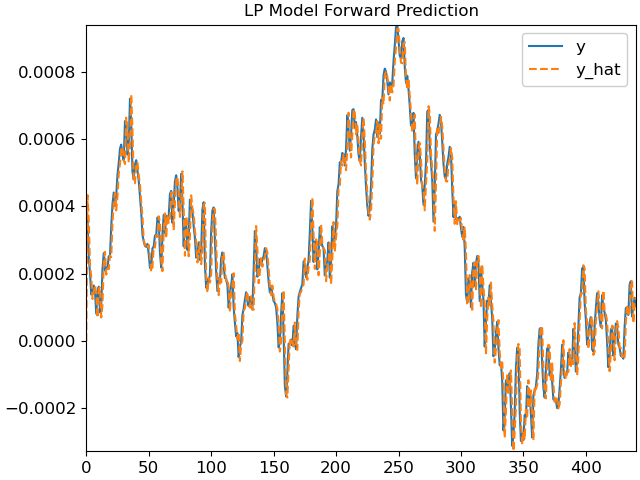# librosa.lpc¶

librosa.lpc(y, order)[source]

Linear Prediction Coefficients via Burg’s method

This function applies Burg’s method to estimate coefficients of a linear filter on `y` of order `order`. Burg’s method is an extension to the Yule-Walker approach, which are both sometimes referred to as LPC parameter estimation by autocorrelation.

It follows the description and implementation approach described in the introduction by Marple. 1 N.B. This paper describes a different method, which is not implemented here, but has been chosen for its clear explanation of Burg’s technique in its introduction.

1

Larry Marple. A New Autoregressive Spectrum Analysis Algorithm. IEEE Transactions on Accoustics, Speech, and Signal Processing vol 28, no. 4, 1980.

Parameters
ynp.ndarray

Time series to fit

orderint > 0

Order of the linear filter

Returns
anp.ndarray of length `order + 1`

LP prediction error coefficients, i.e. filter denominator polynomial

Raises
ParameterError
FloatingPointError
• If `y` is ill-conditioned

Examples

Compute LP coefficients of y at order 16 on entire series

```>>> y, sr = librosa.load(librosa.ex('trumpet'))
>>> librosa.lpc(y, 16)
```

Compute LP coefficients, and plot LP estimate of original series

```>>> import matplotlib.pyplot as plt
>>> import scipy
>>> y, sr = librosa.load(librosa.ex('trumpet'), duration=0.020)
>>> a = librosa.lpc(y, 2)
>>> b = np.hstack([, -1 * a[1:]])
>>> y_hat = scipy.signal.lfilter(b, , y)
>>> fig, ax = plt.subplots()
>>> ax.plot(y)
>>> ax.plot(y_hat, linestyle='--')
>>> ax.legend(['y', 'y_hat'])
>>> ax.set_title('LP Model Forward Prediction')
```